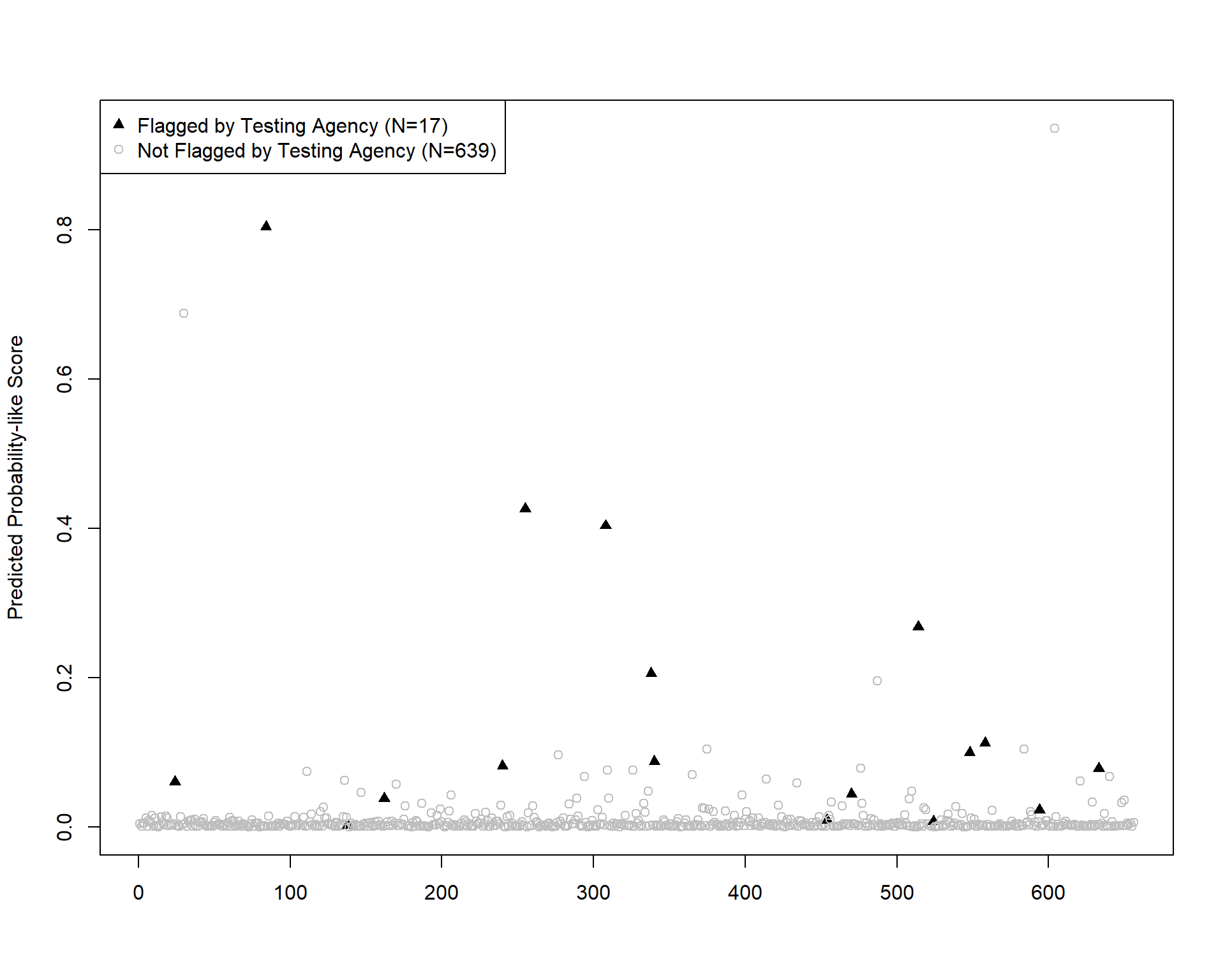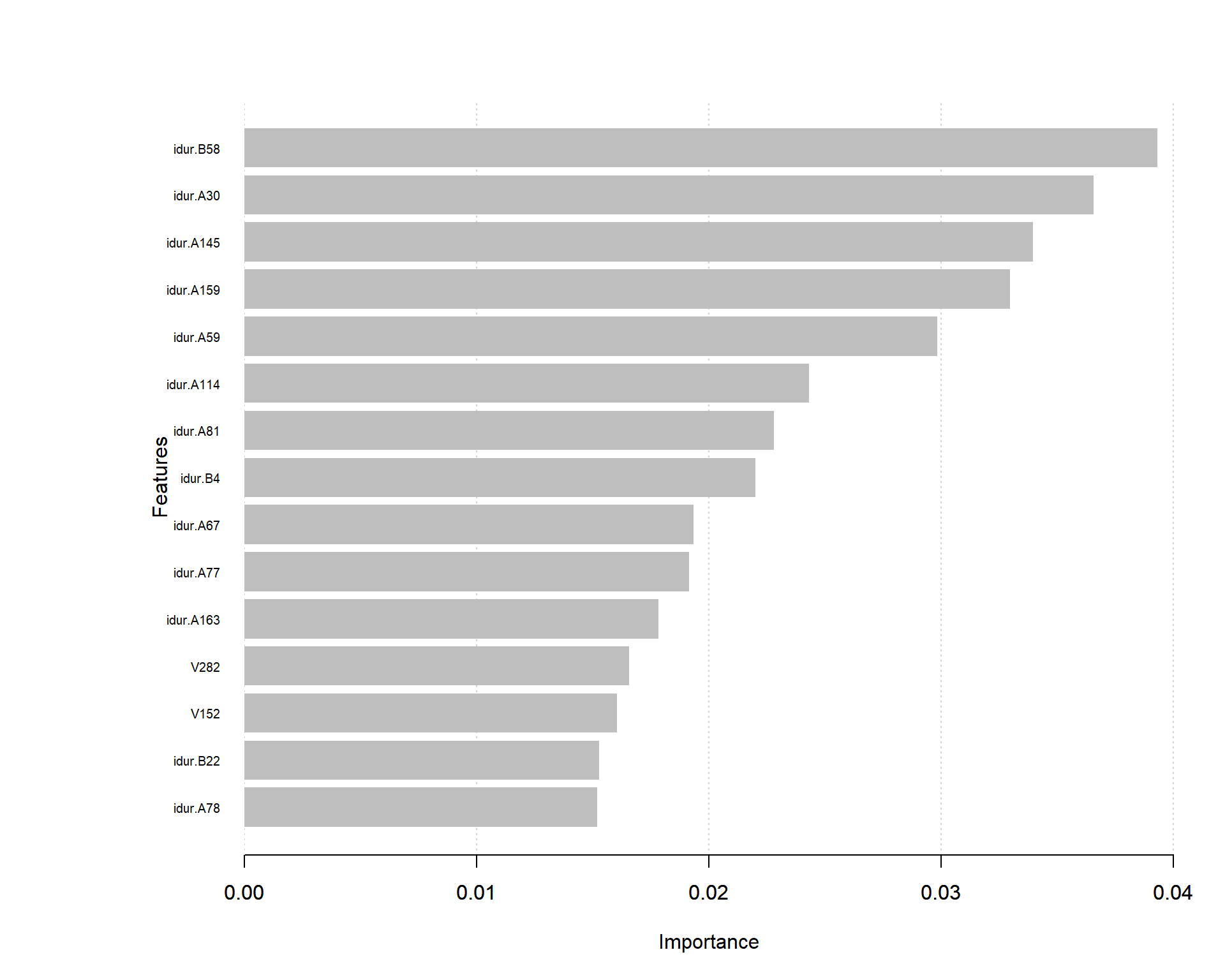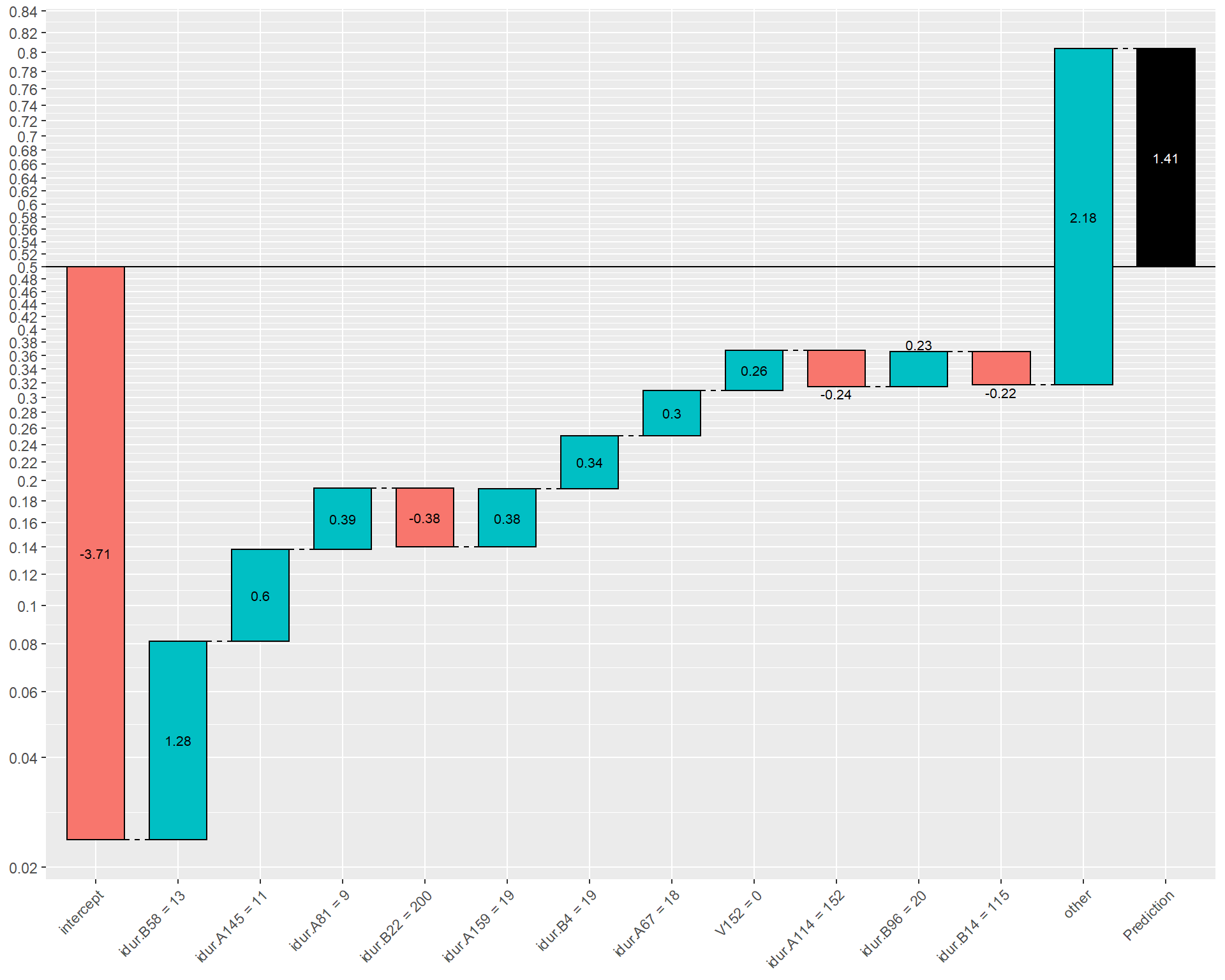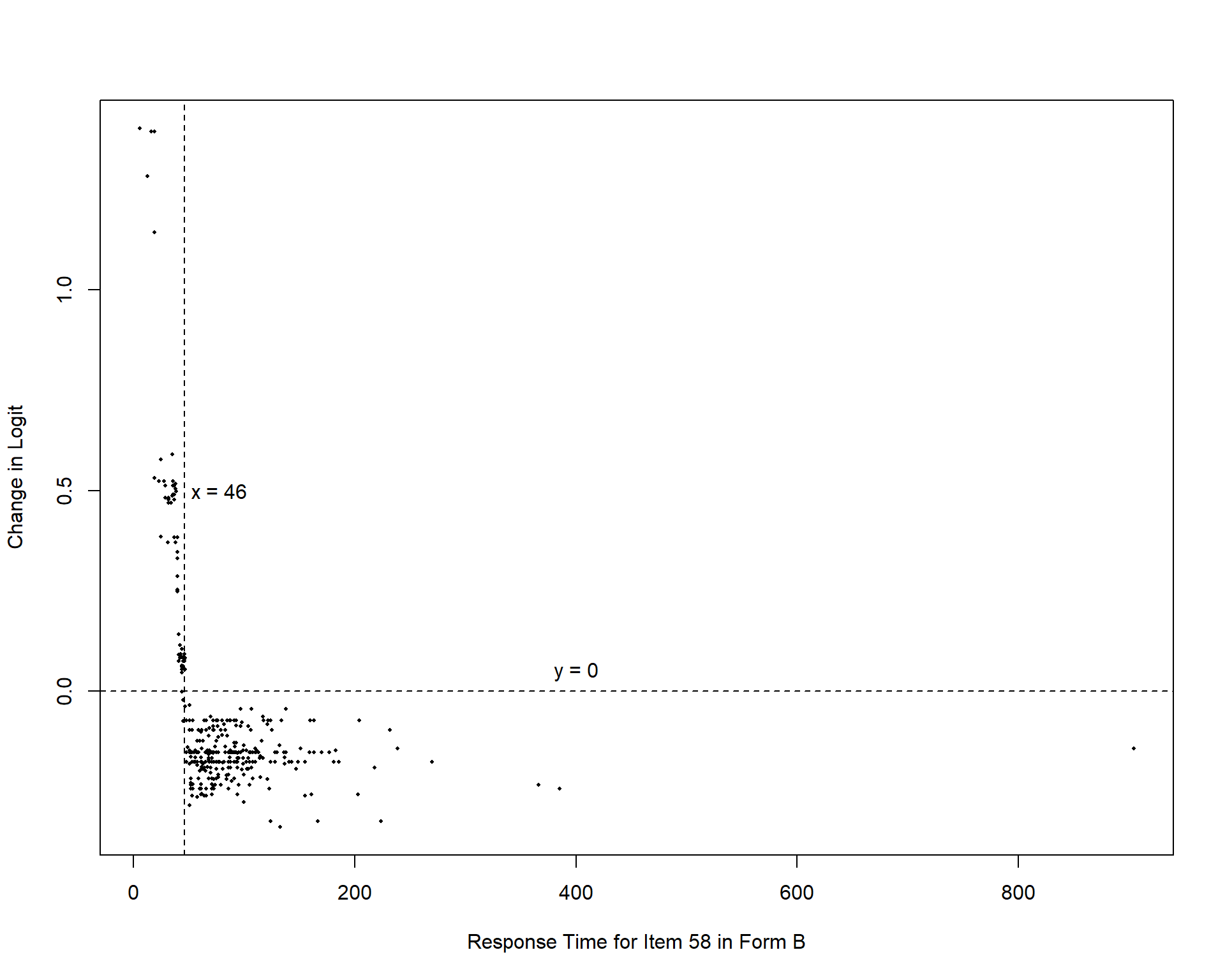# XGBoost Analysis of Real Dataset to Predict Item Preknowledge

This post includes supplemental material to reproduce the real data analysis presented in the recently published EPM paper.

Cengiz Zopluoglu (University of Miami)
04-18-2019

This post includes supplemental material for the real data analysis presented in the EPM paper. Another post that includes the R code to reproduce the numerical illustration in the paper can also be found here, How does XGBoost work?.

The two datasets for the real data analysis in the paper are available from the following links:

Please note that these datasets are made available by Drs. Gregory J. Cizek and James A. Wollack for the chapter I wrote in Handbook of Quantitative Methods for Detecting Cheating on Tests. If you ever decide to use these datasets for any purpose or future publication cite this book as a source of this dataset.


Cizek, G. J., & Wollack, J. A. (Eds.). (2017). Handbook of quantitative methods for detecting cheating on tests. New York, NY: Routledge.

## Datasets

The datasets are obtained by merging two test forms provided by Cizek and Wollack (2017). Each test form had 170 items and shared 87 common items, yielding a total of 253 unique items. Each item was a multiple-choice test item with four nominal options (A, B, C, and D). I recoded each item using something known as one-hot encoding such that each nominal response was represented by four binary indicator variables.

• A = {1,0,0,0}
• B = {0,1,0,0}
• C = {0,0,1,0}
• D = {0,0,0,1}

Therefore, for instance, the first four columns in the dataset are the binary indicator variables for the nominal response given to the first item, the second four columns in the dataset are the binary indicator variables for the nominal response given to the second item, etc. The first 1012 columns correspond to the nominal responses given to all 253 items (4 x 253 = 1012). Then, the following 253 columns (column labels have the prefix idur.) are the response times for all items. For instance, idur.A4 indicates the response time for Item 4 in Form A or idur.B1 indicates the response time for Item 1 in Form B. The last column labeled as Flagged is the outcome variable of interest and indicates whether or not the individual had been identified as a potential cheater by the testing company. These individuals were believed to have prior access to the test items.

Note that, each individual had taken only 170 items in the test form assigned. Therefore, the variables corresponding to 83 items unique to the other test form are missing for each individual, yielding a quite sparse dataset.

The test dataset was created by randomly selecting 20% of the whole dataset, and the remaining 80% was treated as the training dataset. Another thing to keep in mind is that the variables should be recognized as ‘numeric’ not as ‘integer’ in R. I don’t know the reason but I realized it made a slight difference in performance.


# Import Data

dim(train)

 2624 1266


dim(test)

  656 1266

str(train)

'data.frame':   2624 obs. of  1266 variables:
$V1 : int 1 1 0 0 0 1 1 0 0 0 ...$ V2       : int  0 0 0 0 0 0 0 0 1 0 ...
$V3 : int 0 0 0 0 0 0 0 1 0 1 ...$ V4       : int  0 0 1 1 1 0 0 0 0 0 ...
$V5 : int 1 0 0 0 1 0 1 0 1 0 ...$ V6       : int  0 0 0 0 0 0 0 0 0 0 ...
$V7 : int 0 0 1 0 0 1 0 0 0 0 ...$ V8       : int  0 1 0 1 0 0 0 1 0 1 ...
$V9 : int 0 0 0 0 1 0 0 0 0 0 ...$ V10      : int  1 1 1 1 0 0 1 1 0 1 ...
$V11 : int 0 0 0 0 0 0 0 0 1 0 ...$ V12      : int  0 0 0 0 0 1 0 0 0 0 ...
$V13 : int 1 0 1 0 0 0 0 0 0 0 ...$ V14      : int  0 1 0 0 1 0 0 0 0 1 ...
$V15 : int 0 0 0 1 0 0 0 0 0 0 ...$ V16      : int  0 0 0 0 0 1 1 1 1 0 ...
$V17 : int 0 0 0 0 0 0 0 1 0 0 ...$ V18      : int  0 0 1 1 0 0 0 0 0 1 ...
$V19 : int 1 1 0 0 1 1 0 0 0 0 ... ....  str(test)  'data.frame': 656 obs. of 1266 variables:$ V1       : int  0 0 0 1 0 0 0 0 0 0 ...
$V2 : int 0 0 0 0 0 0 1 0 0 0 ...$ V3       : int  1 1 1 0 0 1 0 1 1 1 ...
$V4 : int 0 0 0 0 1 0 0 0 0 0 ...$ V5       : int  1 1 1 1 1 1 1 1 0 1 ...
$V6 : int 0 0 0 0 0 0 0 0 1 0 ...$ V7       : int  0 0 0 0 0 0 0 0 0 0 ...
$V8 : int 0 0 0 0 0 0 0 0 0 0 ...$ V9       : int  0 0 0 0 0 0 0 0 0 0 ...
$V10 : int 1 1 1 1 1 1 1 1 1 1 ...$ V11      : int  0 0 0 0 0 0 0 0 0 0 ...
$V12 : int 0 0 0 0 0 0 0 0 0 0 ...$ V13      : int  1 0 0 0 1 1 0 0 0 1 ...
$V14 : int 0 1 1 1 0 0 1 1 1 0 ...$ V15      : int  0 0 0 0 0 0 0 0 0 0 ...
$V16 : int 0 0 0 0 0 0 0 0 0 0 ...$ V17      : int  1 1 1 1 1 1 0 1 1 0 ...
$V18 : int 0 0 0 0 0 0 0 0 0 0 ...$ V19      : int  0 0 0 0 0 0 0 0 0 0 ...
....

# Convert the variable type to numeric for all variables

for(i in 1:1266) { train[,i] = as.numeric(train[,i])}
for(i in 1:1266) {  test[,i] = as.numeric(test[,i])}

## Fitting the XGBoost model and evaluating the model performance


# install.packages('xgboost')
# install.packages(pROC)

require(xgboost)
require(pROC)

# Create the xgb.DMatrix objects

dtrain <- xgb.DMatrix(data = data.matrix(train[,1:1265]), label=train$Flagged) dtest <- xgb.DMatrix(data = data.matrix(test[,1:1265]), label=test$Flagged)

# Fit the XGBoost model with the tuned parameters

# Tuning the parameters is a whole different story, and I tried to explain it in the paper.
# Maybe, I can do another post about it, but there is already a lot of resources on the web about it.

watchlist <- list(train=dtrain, test=dtest)

bst <- xgb.train(data              = dtrain,
nround            = 10000,
eta               = .05,
min_child_weight  = 1.2,
max_depth         = 3,
gamma             = 0,
max_delta_step    = 0.5,
subsample         = 1,
colsample_bytree  = 1,
lambda            = 0.6,
alpha             = 0.3,
scale_pos_weight  = 1,
num_parallel_tree = 1,
objective         = 'binary:logistic',
eval_metric       = 'rmse',
watchlist         = watchlist,
early_stopping_round  = 1000,
predict = TRUE,
seed=123)

 train-rmse:0.494065 test-rmse:0.494136
Multiple eval metrics are present. Will use test_rmse for early stopping.
Will train until test_rmse hasn't improved in 1000 rounds.

 train-rmse:0.488163 test-rmse:0.488282
 train-rmse:0.482251 test-rmse:0.482446
 train-rmse:0.476363 test-rmse:0.476622
 train-rmse:0.470485 test-rmse:0.470815
 train-rmse:0.464628 test-rmse:0.465032
 train-rmse:0.458775 test-rmse:0.459285
 train-rmse:0.452949 test-rmse:0.453562
 train-rmse:0.447161 test-rmse:0.447846
    train-rmse:0.441386 test-rmse:0.442188
    train-rmse:0.435633 test-rmse:0.436518
    train-rmse:0.429931 test-rmse:0.430891
    train-rmse:0.424241 test-rmse:0.425326
    train-rmse:0.418598 test-rmse:0.419755
    train-rmse:0.412991 test-rmse:0.414238
    train-rmse:0.407403 test-rmse:0.408735
    train-rmse:0.401869 test-rmse:0.403319
....

# Predict the outcome for the test dataset based on the model

test$prob <- predict(bst,dtest) # Create the plot for predicted scores for flagged and unflagged examinees plot(test$prob,
pch=ifelse(test$Flagged==0,1,17), col=ifelse(test$Flagged==0,'gray','black'),
xlab='',
ylab='Predicted Probability-like Score',
main = '')

legend('topleft',
c('Flagged by Testing Agency (N=17)','Not Flagged by Testing Agency (N=639)'),
pch=c(17,1),
col=c('black','gray'))# AUROC

auc(test$Flagged,test$prob)

Area under the curve: 0.9278

# Determine the thresholds for the false positive rates of 0.05 and 0.01

th = quantile(test[test$Flagged==0,]$prob,c(.95,.99))
th

95%        99%
0.03151065 0.07820591 

# Confusion matrix corresponding to .05 Type I error rate

table(test$Flagged,test$prob>th)


FALSE TRUE
0   607   32
1     4   13

# Type I error = 32/639 = 0.050
# Power        = 13/17  = 0.765
# Precision    = 13/45  = 0.288

# Confusion matrix corresponding to .01 Type I error rate

table(test$Flagged,test$prob>th)


FALSE TRUE
0   632    7
1     7   10

# Type I error = 7/639  = 0.011
# Power        = 10/17  = 0.588
# Precision    = 10/17  = 0.588

## Feature importance

The code and figure below provides the most important 15 features in this model. On the x-axis, importance score indicates how valuable a feature is in the construction of boosted trees within the model. The importance score is calculated for a single tree by the amount that each attribute split point improves the performance (as measured by gain weighted by the number of observations in each leaf) and then averaged across all trees within the model. On the y-axis, there are labels for features. For instance, the most important feature turns out to be the response time for Item 58 in Form B (idur.B58), and the second most important feature is the response time for Item 30 in Form A (idur.A30). This plot is important as it may help contextualize why a certain individual’s predicted probability is high after combining the information presented in the next section.


imp = xgb.importance(model=bst)

xgb.plot.importance(importance_matrix = imp,
top_n = 15,
xlim=c(0,.04),
xlab='Importance',
ylab='Features')## Interpreting the XGBoost prediction for an individual


#install.packages("xgboostExplainer")

require(xgboostExplainer)

explainer = buildExplainer(xgb.model    = bst,
trainingData = dtrain,
type         = 'binary',
base_score   = 0.5,
trees_idx    = NULL)

# Breakdown of model prediction for Examinee 84 into the impact of each individual feature on the logit scale

showWaterfall(xgb.model     = bst,
explainer     = explainer,
DMatrix       = dtest,
data.matrix   = data.matrix(test[,1:1265]),
idx           = 84,
type          = 'binary',
threshold     = 0.2)The model predicted a probability of 0.804 for Examinee 84 to have item preknowledge. Suppose one wants to understand why Examinee 84 has a high probability of item preknowledge. The code above provides a breakdown of this prediction into the impact of each individual feature on the logit scale. The first bar is the intercept displaying the logit value of -3.71 with a corresponding probability of 0.024. The second bar indicates that the base prediction changes by 1.28 based on the response time of Examinee 84 on Item 58 in Form B and the new prediction is now -2.43 with a corresponding probability of 0.081. Similarly, the third bar indicates that the prediction changes by 0.6 based on the response time of Examinee 84 on Item 145 in Form A and the new prediction becomes -1.83 with a corresponding probability of 0.138. This continues until each feature makes a negative or positive contribution to the prediction based on Examinee 84’s response data, and the final prediction for Examinee 84 is 1.41 corresponding to a probability of 0.804.

Notice that the short response time on Items 4, 58, and 96 in Form B and Items 67, 81, 145, and 159 in Form A makes a positive contribution that increases the probability, while long response time on Items 14 and 22 in Form B and Item 114 in Form A makes a negative contribution that decreases the probability. It is no coincidence that most of these features can actually be seen in the figure above among the most important 15 features. Only features with an absolute impact larger than .2 are displayed here for the sake of simplicity. The collective contribution of all other features is displayed as ‘other’ on the x-axis.

## Analyzing the relationship between a feature and the predicted outcome

We can also show how a certain value of a feature affects the logit as shown below. Each point in the figure below is an examinee in the test data set. The x-axis representsthe response time of the examinees in the test data set for Item 58 in Form B and the y-axis represents how much the predicted logit changes for each data point.


pred.breakdown = explainPredictions(xgb.model = bst,
explainer = explainer,
data      = dtest)

plot(test[,'idur.B58'],
as.matrix(pred.breakdown[,'idur.B58'])[,1],
cex=0.4,
pch=16,
xlab = 'Response Time for Item 58 in Form B',
ylab = 'Change in Logit')

abline(h=0,lty=2)
abline(v=46,lty=2)

text(400,.05,'y = 0')
text(78,.5,'x = 46')### Citation

Zopluoglu (2019, April 18). Cengiz Zopluoglu: XGBoost Analysis of Real Dataset to Predict Item Preknowledge. Retrieved from https://github.com/czopluoglu/website/tree/master/docs/posts/xgboost/
@misc{zopluoglu2019xgboost,
}